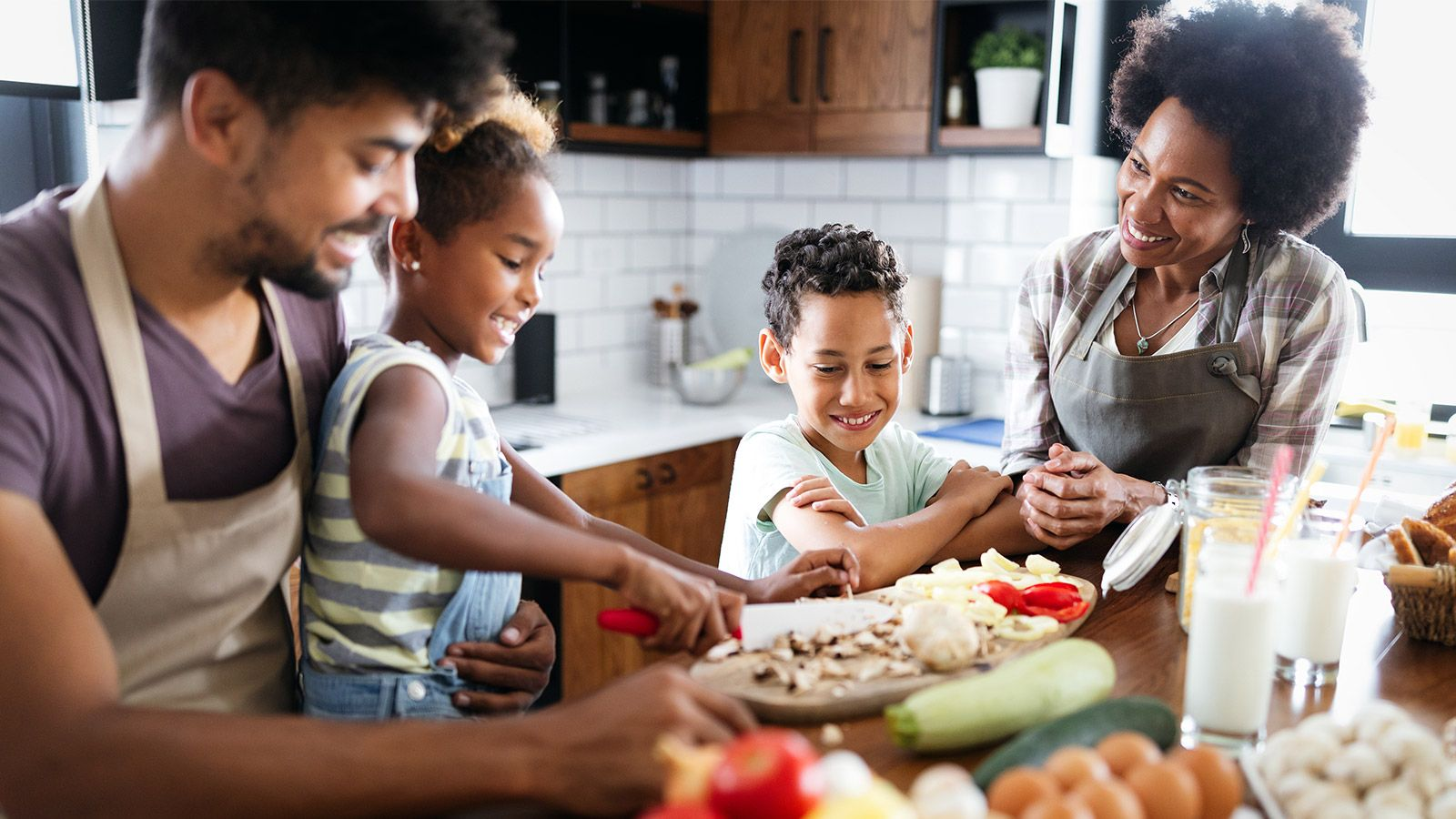# Appliances.self.__wrap_b=(id, ratio, wrapper)=>{ wrapper = wrapper || document.querySelector(`[data-br="\${id}"]`); const container = wrapper.parentElement; const update = (width)=>wrapper.style.maxWidth = width + "px"; // Reset wrapper width wrapper.style.maxWidth = ""; // Get the initial container size const width = container.clientWidth; const height = container.clientHeight; // Synchronously do binary search and calculate the layout let lower = width / 2 - 0.25; let upper = width + 0.5; let middle; if (width) { while(lower + 1 < upper){ middle = Math.round((lower + upper) / 2); update(middle); if (container.clientHeight === height) { upper = middle; } else { lower = middle; } } // Update the wrapper width update(upper * ratio + width * (1 - ratio)); } // Create a new observer if we don't have one. // Note that we must inline the key here as we use `toString()` to serialize // the function. if (!wrapper["__wrap_o"]) { (wrapper["__wrap_o"] = new ResizeObserver(()=>{ self.__wrap_b(0, +wrapper.dataset.brr, wrapper); })).observe(container); } };self.__wrap_b(":Rcp6:",1)

The world’s leading appliance brands partner with Fresco, making it easier than ever to cook delicious food using the appliances you have in your kitchen.

Simply download the app, connect your smart appliances, and we’ll find the perfect recipes to work with them.

## Discover the cross-brand smart kitchen platform.self.__wrap_b=(id, ratio, wrapper)=>{ wrapper = wrapper || document.querySelector(`[data-br="\${id}"]`); const container = wrapper.parentElement; const update = (width)=>wrapper.style.maxWidth = width + "px"; // Reset wrapper width wrapper.style.maxWidth = ""; // Get the initial container size const width = container.clientWidth; const height = container.clientHeight; // Synchronously do binary search and calculate the layout let lower = width / 2 - 0.25; let upper = width + 0.5; let middle; if (width) { while(lower + 1 < upper){ middle = Math.round((lower + upper) / 2); update(middle); if (container.clientHeight === height) { upper = middle; } else { lower = middle; } } // Update the wrapper width update(upper * ratio + width * (1 - ratio)); } // Create a new observer if we don't have one. // Note that we must inline the key here as we use `toString()` to serialize // the function. if (!wrapper["__wrap_o"]) { (wrapper["__wrap_o"] = new ResizeObserver(()=>{ self.__wrap_b(0, +wrapper.dataset.brr, wrapper); })).observe(container); } };self.__wrap_b(":R5cp396:",1)## Fresco mobile appself.__wrap_b=(id, ratio, wrapper)=>{ wrapper = wrapper || document.querySelector(`[data-br="\${id}"]`); const container = wrapper.parentElement; const update = (width)=>wrapper.style.maxWidth = width + "px"; // Reset wrapper width wrapper.style.maxWidth = ""; // Get the initial container size const width = container.clientWidth; const height = container.clientHeight; // Synchronously do binary search and calculate the layout let lower = width / 2 - 0.25; let upper = width + 0.5; let middle; if (width) { while(lower + 1 < upper){ middle = Math.round((lower + upper) / 2); update(middle); if (container.clientHeight === height) { upper = middle; } else { lower = middle; } } // Update the wrapper width update(upper * ratio + width * (1 - ratio)); } // Create a new observer if we don't have one. // Note that we must inline the key here as we use `toString()` to serialize // the function. if (!wrapper["__wrap_o"]) { (wrapper["__wrap_o"] = new ResizeObserver(()=>{ self.__wrap_b(0, +wrapper.dataset.brr, wrapper); })).observe(container); } };self.__wrap_b(":Rpkfb96:",1)

Delicious meals are just a few steps away.

Get the free app on your phone to discover new recipes for your appliances.